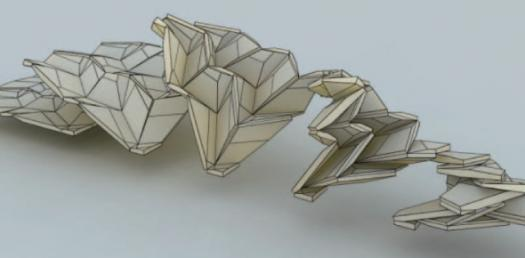# Interesting Quizzes On Rigid Origami

10 Questions | Total Attempts: 142SettingsHave you heard of origami? It is the famous art of folding paper into a figure. On the other hand, rigid origami is an aspect of origami involved with the use of flat rigid sheets joined by hinges. This quiz tests your knowledge of rigid origami. Have fun.

• 1.
A set of rules related to the mathematical principles of paper folding is the?
• A.

Planar axioms

• B.

Mock rules

• C.

Huzita–Hatori axioms

• D.

Duran axioms

• 2.
Maekawa's theorem states that at any vertex the number of valley and mountain folds always differ by?
• A.

One

• B.

Two

• C.

Three

• D.

Zero

• 3.
Kawasaki's theorem states that at any vertex, the sum of all the odd angles adds up to?
• A.

360 degrees

• B.

90 degrees

• C.

270 degrees

• D.

180 degrees

• 4.
In the flat folding of a pure origami, a sheet can never penetrate a?
• A.

Fold

• B.

Pattern

• C.

Crease

• D.

Line

• 5.
The product of principal curvatures of a surface at a point is referred to as?
• A.

Orion plane

• B.

Maekwa curvature

• C.

• D.

Gaussian curvature

• 6.
What is a function that is its own inverse?
• A.

Cipher

• B.

Domain

• C.

Involution

• D.

Quaternion

• 7.
Which is not an example of impossible construction of geometric figures?
• A.

Squaring the circle

• B.

• C.

Doubling the cube

• D.

Angle trisection

• 8.
A rigid fold that has been used to deploy large solar panel arrays for space satellites is the?
• A.

Cut fold

• B.

Napkin fold

• C.

Miura map fold

• D.

Wet fold

• 9.
The problem of whether a square or rectangle of paper can be folded so the perimeter of the flat figure is greater than that of the original square is the?
• A.

Napkin folding problem

• B.

Wet folding

• C.

Fold-and-cut problem

• D.

Galllivan problem

• 10.
Which origami folding allows for a greater range of shapes?
• A.

Wet

• B.

Cut

• C.

Napkin

• D.

Sheet

Related TopicsBack to top